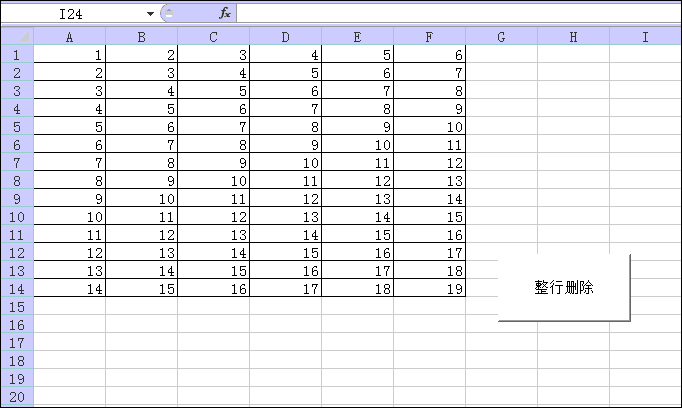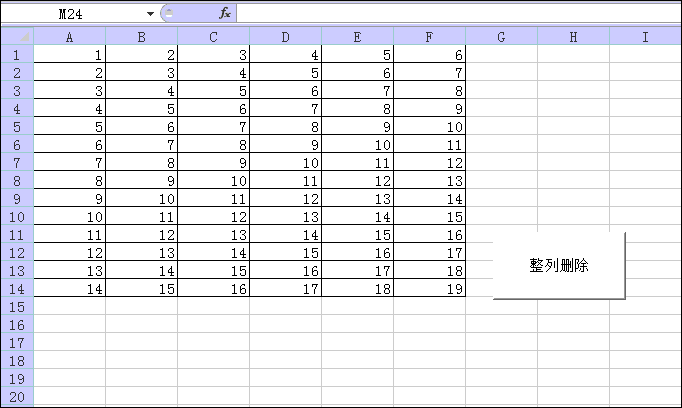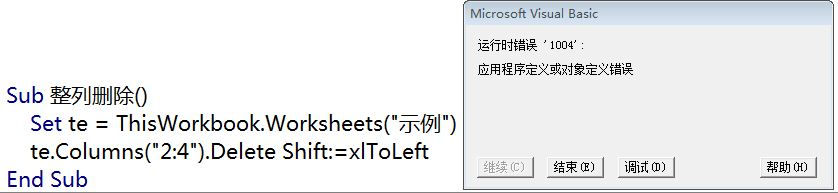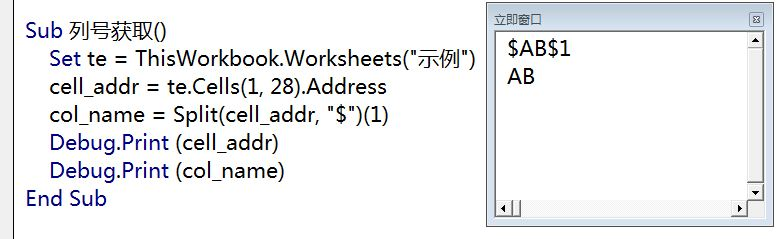• Excel VBA删除行、或单元格
千次阅读
2020-07-21 01:59:16

应用于“应用于”列表中 Range 以外的对象的 Delete 方法。

删除指定的对象。

expression.Delete

表达式 必需。该表达式返回上述对象之一。

应用于 Range 对象的 Delete 方法。

删除指定的对象。

expression.Delete(Shift)

表达式 必需。该表达式返回上述对象之一。Shift   XlDeleteShiftDirection 类型，可选。仅用于 Range 对象。指定如何移动单元格来代替删除的单元格。

 XlDeleteShiftDirection 可为以下 XlDeleteShiftDirection 常量之一。 xlShiftToLeft xlShiftUp 如果省略本参数，则由 Microsoft Graph 根据指定区域的形状来决定如何移动单元格。

## 说明

删除 Point 对象或 LegendKey 对象将删除整个数据系列。

## 示例

以下示例删除数据表上的单元格区域 A1:D10，并将其余单元格左移以填补被删除单元格的位置。

Set mySheet = myChart.Application.DataSheet mySheet.Range("A1:D10").Delete Shift:=xlShiftToLeft

以下示例删除图表标题。

myChart.ChartTitle.Delete

source:VBA Help

更多相关内容
• VBA示例函数之 删除空白，供初学者参考，大牛勿进~~~~~~~
• word、wps中使用vba删除所有表格指定 处理word表格搞得人头大，找时间百度一波写了个函数处理标记删除的问题，根据这一套逻辑还可以实现很多功能，供各位参考。 要点： 双循环遍历所有表表头 InStr函数匹配具体...

### word、wps中使用vba删除所有表格指定列

处理word表格搞得人头大，找时间百度一波写了个函数处理标记删除的问题，根据这一套逻辑还可以实现很多功能，供各位参考。

要点：
双循环遍历所有表表头
InStr函数匹配具体内容（word表格多半有特殊字符，相等匹配较难保证）
在内循环结束后进行删除，否则将出错

用法：
1、定义条件，如 InStr(value1, “学号”)，多条件拼接(如 InStr(value1, “学号”)>0 or InStr(value1, “姓名”) >0)
2、新建宏名range，粘贴内容到vba编辑器
3、执行

效果：代码：



'ver 2.0

Sub range()
'遍历文档所有表格，删除表头第一行含有特定字符串的列
Dim value1 As String
Dim target() As Integer
For i = 1 To ActiveDocument.Tables.Count
Dim targetLen As Integer
targetLen = 0
For j = 1 To ActiveDocument.Tables(i).Columns.Count
value1 = ActiveDocument.Tables(i).Columns(j).Cells(1).range.Text
'这里定义条件，可以定义多个条件
If InStr(value1, "测试") > 0 or InStr(value1, "学号") > 0 Then
targetLen = targetLen + 1
ReDim Preserve target(targetLen)
target(targetLen - 1) = j
Else
End If
Next j
If targetLen <> 0 Then
' MsgBox targetLen
' 修正删除操作带来的列号偏移
Dim shift As Integer
shift = 0
For k = 0 To (targetLen - 1)
' MsgBox target(k)
ActiveDocument.Tables(i).Columns(target(k) - shift).Delete
shift = shift + 1
Next k
End If
Next i
MsgBox ("执行完毕。")
End Sub


使用过程中遇到复合表格遍历报错问题，修改加入错误处理跳过
（注意，因需求变动这里已经改成了匹配第一列，如仍需匹配第一行，请按上一段代码循环条件设置）

'ver 3.0
Dim value1 As String
Dim target() As Integer
For i = 1 To ActiveDocument.Tables.Count
'MsgBox ("遍历表 " & i & "。")
Dim targetLen As Integer
targetLen = 0
For j = 1 To ActiveDocument.Tables(i).Rows.Count
On Error GoTo flag
value1 = ActiveDocument.Tables(i).Rows(j).Cells(1).range.Text
'这里定义条件
If InStr(value1, "test1") > 0 Or InStr(value1, "test2") > 0  Then
targetLen = targetLen + 1
ReDim Preserve target(targetLen)
target(targetLen - 1) = j
Else
End If
Next j
If targetLen <> 0 Then
' MsgBox targetLen
' 修正删除操作带来的列号偏移
Dim shift As Integer
shift = 0
For k = 0 To (targetLen - 1)
' MsgBox target(k)
ActiveDocument.Tables(i).Rows(target(k) - shift).Delete
shift = shift + 1
Next k
End If
flag:
Resume continue
continue:
Next i
MsgBox ("执行完毕。")
End Sub


如需删除而非跳过复合行，则删除代码需换成下列实现形式

 With ActiveDocument
.Tables(1).Cell(2, 2).Delete ShiftCells:=wdDeleteCellsEntireRow
End With

展开全文wps openoffice
• 删除空余： Dim LastColumn As Long, c As Long LastColumn = ActiveSheet.UsedRange.Columns.Count LastColumn = LastColumn + ActiveSheet.UsedRange.Column For c = LastColumn To 1 Step -1 If ...
• sub1.删除工作表所有空行 sub2.删除工作表所有空 sub3.删除选中单列包含指定字符的行 sub4.删除选中单列不含指定字符的行

## sub1.删除工作表所有空行

Sub 删除工作表所有空行()
Dim first_row, last_row, i
first_row = ActiveSheet.UsedRange.Row
last_row = first_row + ActiveSheet.UsedRange.Rows.count - 1
For i = last_row To first_row Step -1   '倒序循环
If WorksheetFunction.CountA(Rows(i)) = 0 Then
Rows(i).Delete  '删除行
End If
Next
End Sub


## sub2.删除工作表所有空列

Sub 删除工作表所有空列()
Dim first_col, last_col, i
first_col = ActiveSheet.UsedRange.Column
last_col = first_col + ActiveSheet.UsedRange.Columns.count - 1
For i = last_col To first_col Step -1   '倒序循环
If WorksheetFunction.CountA(Columns(i)) = 0 Then
Columns(i).Delete  '删除列
End If
Next
End Sub


## sub3.删除选中单列包含指定字符的行

Sub 删除选中单列包含指定字符的行()
'选中单列整列、单列部分都支持
Dim rng As Range, arr, first_row, last_row, first_col, i, j
'--------------------参数填写：arr，指定条件字符串数组；title_row，表头行数
'要删除的字符串数组，空值为删除空单元格，可使用模式匹配
arr = Array("*一", "*三", "*五")
title_row = 1        '表头行数，不执行删除
Set rng = Intersect(ActiveSheet.UsedRange, Selection)  'intersect语句避免选择整列造成无用计算
If rng.Columns.count > 1 Then Debug.Print "仅支持单列": Exit Sub  '仅支持单列，多列则退出
first_row = WorksheetFunction.Max(title_row, rng.Row)  '表头行与选中区域开始行号的大值
last_row = rng.Row + rng.Rows.count - 1  '选中区域结束行号
first_col = rng.Column  '选中区域开始列号

If rng.Row = 1 Then  '选中单列整列
For i = last_row To title_row + 1 Step -1  '倒序循环
For Each j In arr
'只要有一个符合，就删除
If Cells(i, first_col) Like j Then Rows(i).Delete
Next
Next
ElseIf rng.Row > 1 Then  '选中单列部分
For i = last_row To first_row Step -1  '倒序循环
For Each j In arr
If Cells(i, first_col) Like j Then Rows(i).Delete
Next
Next
End If
End Sub


### 举例

A列选中运行sub3后得到C列效果## sub4.删除选中单列不含指定字符的行

Sub 删除选中单列不含指定字符的行()
'选中单列整列、单列部分都支持
Dim rng As Range, arr, first_row, last_row, first_col, i, j, del_if As Boolean
'--------------------参数填写：arr，指定条件字符串数组；title_row，表头行数
'要删除的字符串数组，空值为删除空单元格，可使用模式匹配
arr = Array("*一", "*三", "*五")
title_row = 1        '表头行数，不执行删除
Set rng = Intersect(ActiveSheet.UsedRange, Selection)  'intersect语句避免选择整列造成无用计算
If rng.Columns.count > 1 Then Debug.Print "仅支持单列": Exit Sub  '仅支持单列，多列则退出
first_row = WorksheetFunction.Max(title_row, rng.Row)  '表头行与选中区域开始行号的大值
last_row = rng.Row + rng.Rows.count - 1  '选中区域结束行号
first_col = rng.Column  '选中区域开始列号

If rng.Row = 1 Then   '选中单列整列
For i = last_row To title_row + 1 Step -1  '倒序循环
del_if = True   '初始为删除
For Each j In arr
'只要有一个符合，就不删除
If Cells(i, first_col) Like j Then del_if = False: Exit For
Next
'都不符合，删除
If del_if Then Rows(i).Delete
Next
ElseIf rng.Row > 1 Then  '选中单列部分
For i = last_row To first_row Step -1  '倒序循环
del_if = True    '初始为删除
For Each j In arr
If Cells(i, first_col) Like j Then del_if = False: Exit For
Next
If del_if Then Rows(i).Delete
Next
End If
End Sub


### 举例

A列选中运行sub4后得到C列效果## sub5.删除选中列重复的整行

对于选中多行多列区域，在一行中所有列的内容都重复，则删除该行，仅保留唯一一行，注意区分字母大小写

Sub 选中列去重()
'适用单/多列选中、单/多列部分选中，去重删除整行
Dim rng As Range, dict As Object, first_row, last_row, first_col, last_col, i, j, res
Set rng = Intersect(ActiveSheet.UsedRange, Selection)  'intersect语句避免选择整列造成无用计算
first_row = rng.Row     '选中区域开始行号
last_row = first_row + rng.Rows.count - 1  '选中区域结束行号
first_col = rng.Column  '选中区域开始列号
last_col = first_col + rng.Columns.count - 1  '选中区域结束列号
Set dict = CreateObject("scripting.dictionary")

For i = last_row To first_row Step -1   '倒序循环，避免遗漏
res = ""
For j = first_col To last_col
res = res & CStr(Cells(i, j).Value)
Next
If Not dict.Exists(res) Then  '字典键不存在，新增
dict(res) = ""
Else
Rows(i).Delete  '删除行
End If
Next

End Sub


### 举例

《excel吧提问-多行多列重复数据筛选》

多列去重前选中A-D列，运行sub5，获得结果展开全文excel
• Excel的多行或者多列删除

系统：Windows 7
软件：Excel 2010

• 这个系列集锦了一些常用功能
• 今天说说对多行多列的删除操作
• 重点强调如何表示多行或者多列

数据源形式## Part 1：多行删除

1. 通过RowsRange两种方法都可以
2. 多行使用行号数字来表示，注意需将行号放入双引号中""
Sub 多行删除()
Set te = ThisWorkbook.Worksheets("示例")
'te.Rows("3:5").Delete Shift:=xlUp
te.Range("3:5").Delete Shift:=xlUp
End Sub

执行效果## Part 2：多列删除

1. 通过ColumnsRange两种方法都可以
2. 列号使用字母表示，注意需将行号放入双引号中""
3. 当使用数字表示列号时，报错
Sub 多列删除()
Set te = ThisWorkbook.Worksheets("示例")
te.Columns("B:D").Delete Shift:=xlToLeft
'    te.Range("B:D").Delete Shift:=xlToLeft
End Sub

执行效果列号采用数字报错信息## Part 3：列号的字母表示

1. 在某些情况下我们只有列号的数字表示怎么办（例如Match函数结果）​？
2. 使用Address结合Split函数即可解决该问题
3. 示例中28列对应的列号为AB
Sub 列号获取()
Set te = ThisWorkbook.Worksheets("示例")
Debug.Print (col_name)
End Sub

执行效果以上为本次的学习内容，下回见

如发现有错误，欢迎留言指出。文章定位：“字典查询类”

更多原创请关注微信公众号

扫描二维码，关注公众号
让重复工作一键化，让时间更有意义展开全文Excel
• ## VBA删除或插入行列

千次阅读 2018-10-18 08:54:28
' '当空白行（A行）的下一行也为空白行（B行）时，就会出现A行被删除，B行却上移得到保留 ' '的处理。对于这种情况，我们可以通过多次重复运行Sub（）得到。 ' End If ' Next '方法二： For i = r To 1 Step -1 ...
• VBA删除如下内容，解决思路都不同 删除1的空行（本文要做的） 删除整个区域内的空行 删除整个区域内的空格（这个一般很少有这种需求，用处不大----可用currentregion .specialcells()解决） 下...
• VBA 按照某一进行筛选拆分数据，拆分成多个表或多个Sheet，按某检查重复生成新的Sheet，删除除本表外的所有表
• 代码如下： Sub july234() Dim i As Integer For Each Y In Worksheets X = Y.UsedRange.Rows.Count For i = 1 To X If Cells(i, "...).EntireRow.Delete '在A删除0所在行 End If ...
• 有时候为了做数据透视，但是很多，看起来眼花，不如插入号来的方便 比如第一“序号”变成“A__序号”,27变为AA开头，依次类推。 Private Sub 插入号() i = 1 Do Until Cells(1, i) = "" Cells(1, i) = ...
• 如题，预计打算把黄色标示的行全部一齐删除。 如果数据比较大达到几十万行的话，循环寻找A列为空则比较低效。 若用SpecialCells函数把空白单元格全部找到而且选中，则不需要循环，速度较快。 Sub Test () dim blk, ...excel
• excel表格处理当前工作表时，如果有几行中穿插着空白行，把下面代码复制进去，自动删除掉 Sub DeleteBlankRow() Dim IngFirstRow As Long Dim IngLastRow As Long Dim a As Integer IngFirstRow = ...
• Excel中用VBA实现删除空行和空.docx
• 批量标色表格两列的重复值为不同颜色，以及删除重复项和一
• Sub d() Dim i As Long Dim r As Long i = 2 r = 2 Do While r <= Sheet2.Rows.Count 'Range("K1").EntireColumn.Cells(i, 1).Value = Range("F2").EntireColumn.Cells(r, 1).Value ...EXCEL
• Excel VBA删除指定条件的Series的图例Legend程序人生
• excel 透视表 vba 使用Excel VBA删除数据透视表计算字段 (Remove Pivot Table Calculated Field With Excel VBA)Yesterday, I started out with the best of intentions, planning to get some work done, and find ...java python mysql 大数据 人工智能
• 定义一个普通按钮   Private Sub CommandButton1_Click() ThisWorkbook.Sheets(1).Rows(1)....删除指定行，下面的纪录向上移 Sub Macro1()  Rows("11:11").Select  Selection.Delete Shift:=xlUp End Subexcel Excel EXCEL
• excel vba Excel VBA：将标题切换为数字 (Excel VBA: Switch Column Headings to Numbers) It's Friday, and your brain is almost full, but let's try to cram a little bit of Excel VBA in there before ...excel python java ubuntu github
• 源数据及要求：删除黄忠和庞统的各一行重复数据，两个孙权因为班级不同，保留 数据结果展示： 代码解析： Sub DeleteSameRow1() '删除所有重复行，保留唯一值 Dim LastRow As Long Dim i, k, n As Long ...
• 我们依旧按下熟悉的alt+f11进入vbe编辑器，新建一个模块输入代码：Sub 提取唯一值()Dim Rng As RangeDim Str As StringDim EndRowNo As IntegerEndRowNo = Range("A1").End(xlDown).Row '找出A最大行数For Each ...
• Sub Del() num = ActiveSheet.UsedRange.Rows.Count() For i = 1 To num If Cells(i, 9) = "" Then Rows(i).Delete End If Next End Sub
• 工作中经常遇到一些软件导出的表格，其中的各级小计分布在不同的，“小计”二字之间有的还加入了数量不等的空格，实际统计时这些小计行在其中非常碍事，于是得想个办法快速删掉它。 提示：删除内容有风险，请谨慎...后端
• 假如某一包含多种重复单元，但是重复的单元对应的其它却不相同，需求便是指定某一，寻找其中相同的元素，对两行元素进行合并。如下图所示的源数据， 可以看到，该Excel表格的A有很多重复项，对他们进行合并...
• 功能：可以一次选择很多个Excel文件，删除设定的文件(A~Z可同时选择多) 工具简易操作说明书 1. 安装 无需安装可直接运行… 2. 运行“XXX”，如下图； 3. 设置 4. 选择文件(可以同时选择多个文件) 双击显示...
• Sub chaifenshuju() Dim sht As Worksheet Dim k, i, j As Integer Dim irow As Integer ...l = InputBox("请输入你要按哪分") '删除无意义的表 Application.DisplayAlerts = False If Sheets.Count &...excel
• 做了一个简单的Excel文件，有一些vba脚本，如果用户随便删除整行/整列会出错。所以，想在Worksheet_Change事件判断当用户进行删除整行或整列操作时，弹一个yes/no框，若用户选择no，则终止删除操作。谢谢。excel 脚本
• ridx = 3 '我要清理的内容是从“清理邮箱”工作表的第3行第5开始的，因此ridx起始值为3，下面的所有标也都是5 Do While w.Cells(ridx, 5) <> "" org_email = VBA.Trim(w.Cells(ridx, 5)) '清理内部空格之前先把...经验分享...